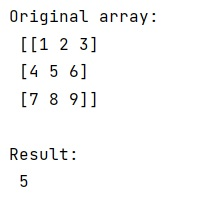# Array slice with comma

Learn about the slicing in numpy array and the significance of comma in slicing.
Submitted by Pranit Sharma, on January 21, 2023

NumPy is an abbreviated form of Numerical Python. It is used for different types of scientific operations in python. Numpy is a vast library in python which is used for almost every kind of scientific or mathematical operation. It is itself an array which is a collection of various methods and functions for processing the arrays.

## NumPy Array – Slicing with comma

While slicing an array, the first number is started, the next is the end, and the last is a step, but there is a comma after the end number and we need to understand the significance of this comma.

The comma in slicing is used to extract a specific column from a 2D array. Old slicing techniques generally involve the following code pattern: X[Y]

Which either calls __getitem__, or __setitem__, now the value which we pass inside slicing (here, Y) has a comma in it, and python will pass a tuple in return.

Let us understand with the help of an example,

## Python code to demonstrate the example of array slice with comma

```# Import numpy
import numpy as np

# Creating a numpy array
arr = np.array([[ 1, 2, 3],[ 4, 5, 6],[ 7, 8, 9]])

# Display original array
print("Original array:\n",arr,"\n")

# Slicing the array using comma
res = arr[1,1]

# Display result
print("Result:\n",res)
```

Output: